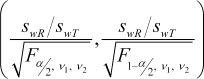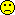## LaTeX, MathML, PNGs [General Sta­tis­tics]

Hi Shuanghe,

congratulations for mastering the nested lists in your post. I’m impressed!

» 9. It seems LaTeX expression can not be used to write math equation here.

Correct.

» Is it possible to implement it?

Theoretically yes.
• It would be possible to add GeSHi which was also used by Wikipedia. In version 2 of the forum’s scripts it turned out that the server-load can be extreme (though fine for distributed sites like WP). It lead to a work­around almost doubling the size of the MySQL-database (one table containing original posts and another one linking to rendered PNGs; example-post). I didn’t like that and decided to stay with the v1.x-branch.
• Another – desirable – option would allow to embed MathML in (X)HTML. Currently the support by browsers is limited (see here; unfortunately 11.1% of users of the forum still on IE).
• Client-side solutions (KaTeX, MathQuill, MathJaxSHJS, …) are a not so nice because some com­pa­nies’ IT-folk restrict JavaScript on user’s machines.
If you enjoy the “beauty” of formulas:⇒ DVI ⇒ Ghostscript ⇒ grayscale (4 or 8 bit) PNG with alpha-trans­parency en­abled and upload/link the file here. Example:Drawbacks: If images are dis­abled in the browser only the image’s attribute alt="[image]" is displayed. Ge­SHi would come up with alt="\left ( \frac{s_{wR} / s_{wT} } {\sqrt{F_{\alpha/2}, \quad {\nu_1}, \quad {\nu_2}}}, \frac{s_{wR} / s_{wT} } {\sqrt{F_{1-\alpha/2}, \quad {\nu_1}, \quad {\nu_2}}} \right )". The former is non-informative and the latter confusing to anybody not familiar with the syntax of AMS.

BTW, simple formulas in the forum can be constructed by means of UTF-8 characters and BB-Codes, e.g.,

CVw = √σ²w − 1

» Since there are so many members here …

Don’t overestimate that. This year so far only 122 of them were active (at least one post). The forum is a rather exclusive club; ten nerds (0.1%) are guilty for 55% of all posts.

» … and some times one has to write complicate formula it might be a good idea to be able to write equations in LaTeX.

I’m afraid users in the forum being knowledgable ofare in the minority… BTW, I once wrote a manu­script in MiKTEX according to the publisher’s templates only to learn that they required bloody M\$-Word in the mean­time. Although I used MathType don’t ask me how the paper looked at the end.Edit: With the MathJax library (installed June 2019):
$$\left(\frac{s_{wR}/s_{wT}} {\sqrt{F_{\alpha/2},\quad{\nu_1},\quad{\nu_2}}},\frac{s_{wR}/s_{wT} }{\sqrt{F_{1-\alpha/2},\quad{\nu_1},\quad{\nu_2}}}\right)$$
$$C{V_w}=\sqrt{{e^{\sigma _w^2}}-1}$$

Dif-tor heh smusma 🖖
Helmut SchützThe quality of responses received is directly proportional to the quality of the question asked. 🚮
Science QuotesIng. Helmut Schütz